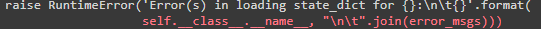I train and save the model I created with collab_learner with fit_one_cycle.
Then I try to load it again and produce predictions with another test data.

this is the part where i build and train the model

data_collab = CollabDataBunch.from_df(ratingsdf)
learn2 = collab_learner(data_collab, n_factors=40, y_range=y_range, wd=0.1)
learn2.fit_one_cycle(10, 5.5e-6)
allLearns = learn2.save(Path('/content/models/trained_model_c'))

This is the part that gives the error. (test1_learner.load)

test1_dataframe = pd.DataFrame(test1_df, columns=['user', 'item', 'rating'])

test1_data = CollabDataBunch.from_df(ratingsdf, test=test1_dataframe, seed=42)

test1_learner = collab_learner(test1_data, n_factors=40, y_range=y_range, wd=0.1)

the error is like this:

ps: first part and second part are have a same size.

thanks in advance for the help…

Hi Eliftugcea
Given the dictionary message I think the save of the data is adding extra characters which accounts for the 1656 verses 1646 numbers.
Regards ConwynHi Conwyn,
Thank you for your reply and attention. Actually I solved the problem. I think there was more than one mistake. (Probably still exists, I’m a beginner). First I used “export” instead of “save” to save the model.
learn.export()

I uploaded the saved “pkl” file as